# Chapter 4 Line Graphs

Line graphs are typically used for visualizing how one continuous variable, on the y-axis, changes in relation to another continuous variable, on the x-axis. Often the x variable represents time, but it may also represent some other continuous quantity, for example, the amount of a drug administered to experimental subjects.

As with bar graphs, there are exceptions. Line graphs can also be used with a discrete variable on the x-axis. This is appropriate when the variable is ordered (e.g., “small”, “medium”, “large”), but not when the variable is unordered (e.g., “cow”, “goose”, “pig”). Most of the examples in this chapter use a continuous x variable, but we’ll see one example where the variable is converted to a factor and thus treated as a discrete variable.

## 4.1 Making a Basic Line Graph

### 4.1.1 Problem

You want to make a basic line graph.

### 4.1.2 Solution

Use `ggplot()` with `geom_line()`, and specify which variables you mapped to x and y (Figure 4.1):

``````ggplot(BOD, aes(x = Time, y = demand)) +
geom_line()``````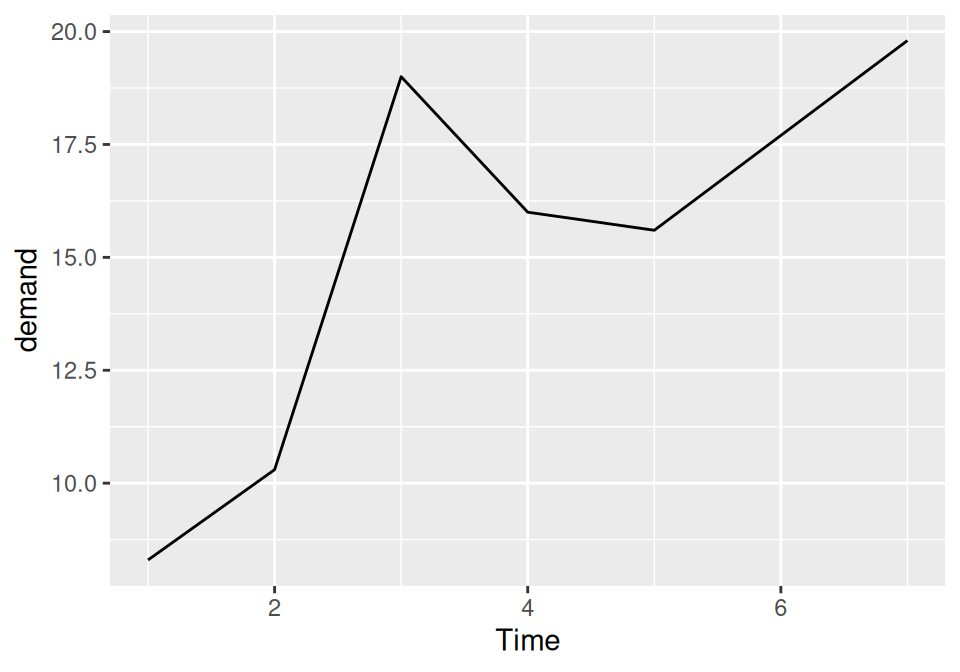Figure 4.1: Basic line graph

### 4.1.3 Discussion

In this sample data set, the x variable, Time, is in one column and the y variable, demand, is in another:

``````BOD
#>   Time demand
#> 1    1    8.3
#> 2    2   10.3
#> 3    3   19.0
#> 4    4   16.0
#> 5    5   15.6
#> 6    7   19.8``````

Line graphs can be made with discrete (categorical) or continuous (numeric) variables on the x-axis. In the example here, the variable demand is numeric, but it could be treated as a categorical variable by converting it to a factor with `factor()` (Figure 4.2). When the x variable is a factor, you must also use `aes(group=1)` to ensure that ggplot knows that the data points belong together and should be connected with a line (see Recipe 4.3 for an explanation of why group is needed with factors):

``````BOD1 <- BOD  # Make a copy of the data
BOD1\$Time <- factor(BOD1\$Time)

ggplot(BOD1, aes(x = Time, y = demand, group = 1)) +
geom_line()``````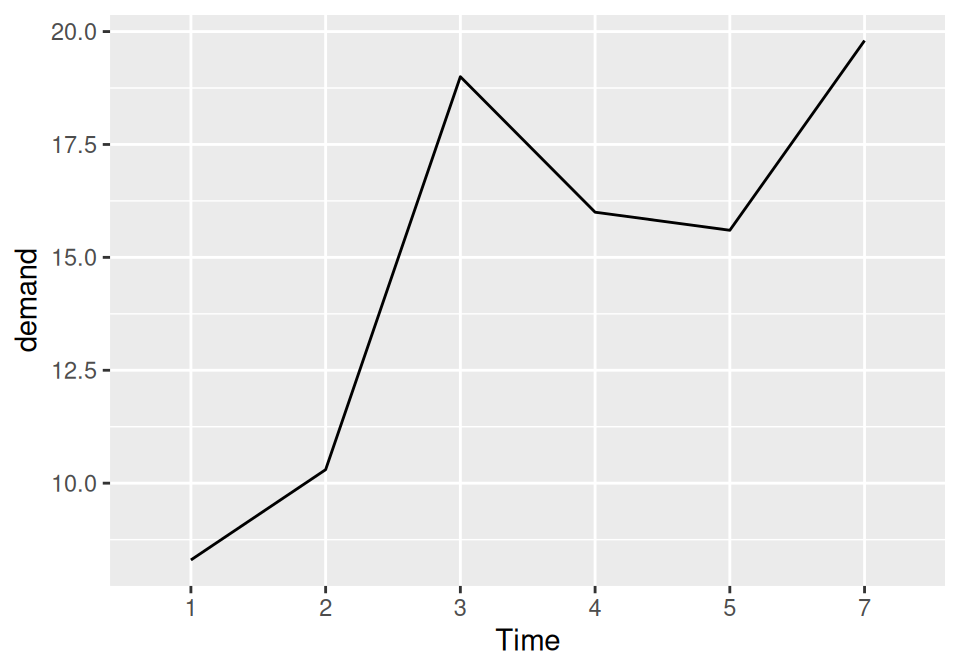Figure 4.2: Basic line graph with a factor on the x-axis (notice that no space is allocated on the x-axis for 6)

In the `BOD` data set there is no entry for `Time = 6`, so there is no level 6 when `Time` is converted to a factor. Factors hold categorical values, and in that context, 6 is just another value. It happens to not be in the data set, so there’s no space for it on the x-axis.

With ggplot2, the default y range of a line graph is just enough to include the y values in the data. For some kinds of data, it’s better to have the y range start from zero. You can use `ylim()` to set the range, or you can use `expand_limits()` to expand the range to include a value. This will set the range from zero to the maximum value of the demand column in `BOD` (Figure 4.3):

``````# These have the same result
ggplot(BOD, aes(x = Time, y = demand)) +
geom_line() +
ylim(0, max(BOD\$demand))

ggplot(BOD, aes(x = Time, y = demand)) +
geom_line() +
expand_limits(y = 0)``````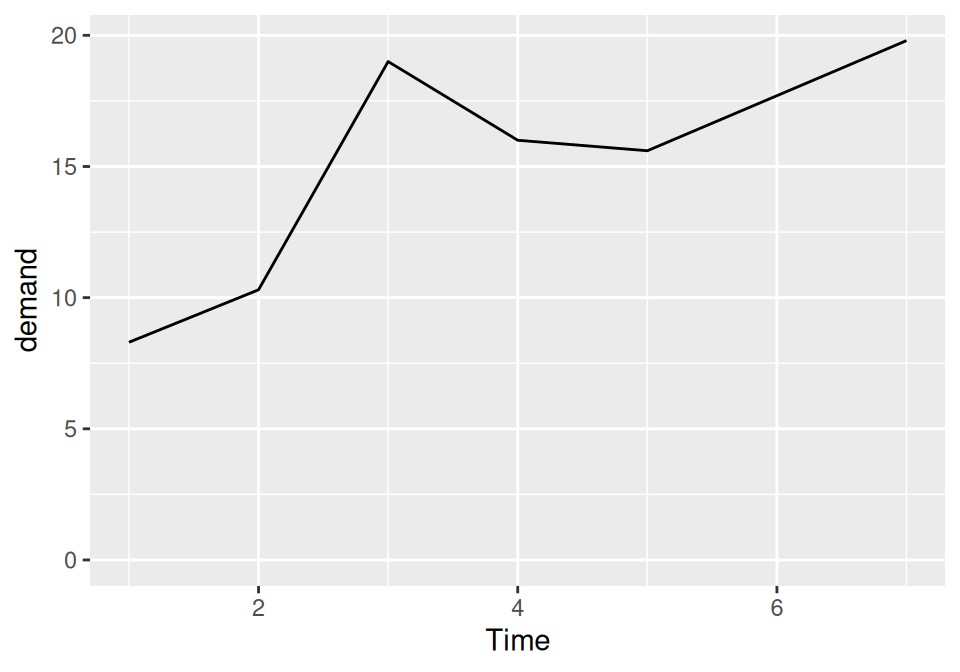Figure 4.3: Line graph with manually set y range

See Recipe 8.2 for more on controlling the range of the axes.

## 4.2 Adding Points to a Line Graph

### 4.2.1 Problem

You want to add points to a line graph.

### 4.2.2 Solution

Add `geom_point()` (Figure 4.4):

``````ggplot(BOD, aes(x = Time, y = demand)) +
geom_line() +
geom_point()``````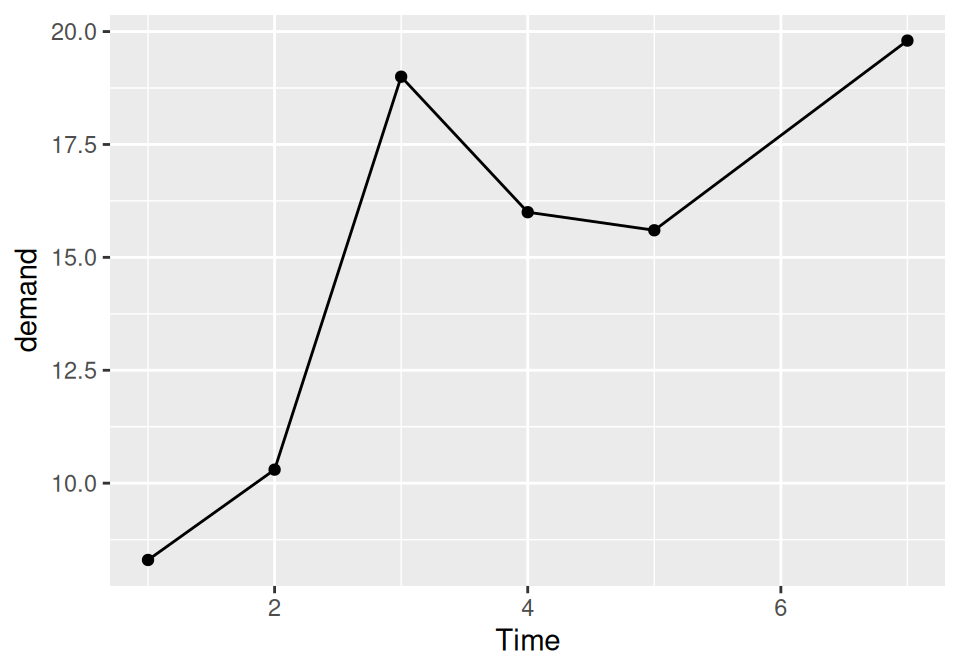Figure 4.4: Line graph with points

### 4.2.3 Discussion

Sometimes it is useful to indicate each data point on a line graph. This is helpful when the density of observations is low, or when the observations do not happen at regular intervals. For example, in the `BOD` data set there is no entry for `Time=6`, but this is not apparent from just a bare line graph (compare Figure 4.3 with Figure 4.4).

In the `worldpop` data set, the intervals between each data point are not consistent. In the far past, the estimates were not as frequent as they are in the more recent past. Displaying points on the graph illustrates when each estimate was made (Figure 4.5):

``````library(gcookbook) # Load gcookbook for the worldpop data set

ggplot(worldpop, aes(x = Year, y = Population)) +
geom_line() +
geom_point()

# Same with a log y-axis
ggplot(worldpop, aes(x = Year, y = Population)) +
geom_line() +
geom_point() +
scale_y_log10()``````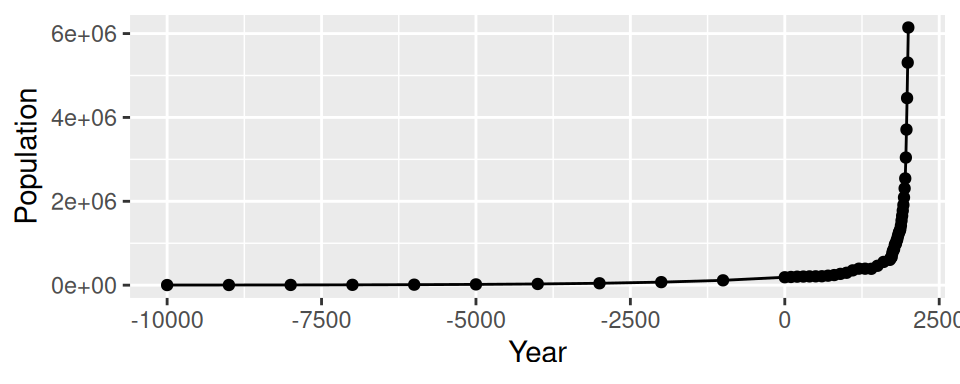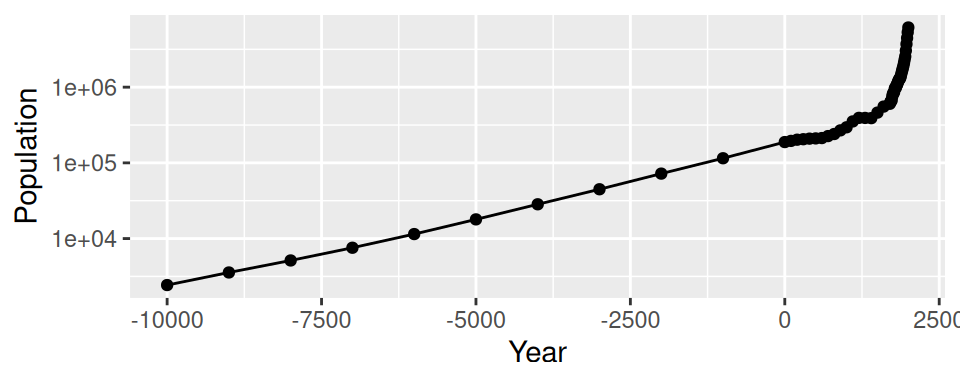Figure 4.5: Top: points indicate where each data point is; bottom: the same data with a log y-axis

With the log y-axis, you can see that the rate of proportional change has increased in the last thousand years. The estimates for the years before 0 have a roughly constant rate of change of 10 times per 5,000 years. In the most recent 1,000 years, the population has increased at a much faster rate. We can also see that the population estimates are much more frequent in recent times–and probably more accurate!

To change the appearance of the points, see Recipe 4.5.

## 4.3 Making a Line Graph with Multiple Lines

### 4.3.1 Problem

You want to make a line graph with more than one line.

### 4.3.2 Solution

In addition to the variables mapped to the x- and y-axes, map another (discrete) variable to colour or linetype, as shown in Figure 4.6:

``````library(gcookbook) # Load gcookbook for the tg data set

# Map supp to colour
ggplot(tg, aes(x = dose, y = length, colour = supp)) +
geom_line()

# Map supp to linetype
ggplot(tg, aes(x = dose, y = length, linetype = supp)) +
geom_line()``````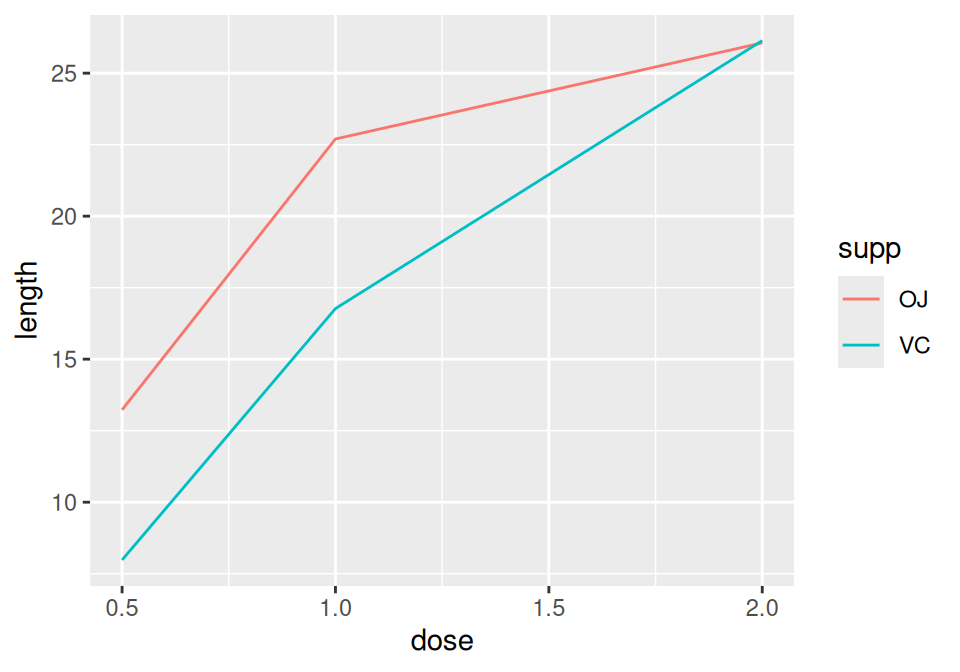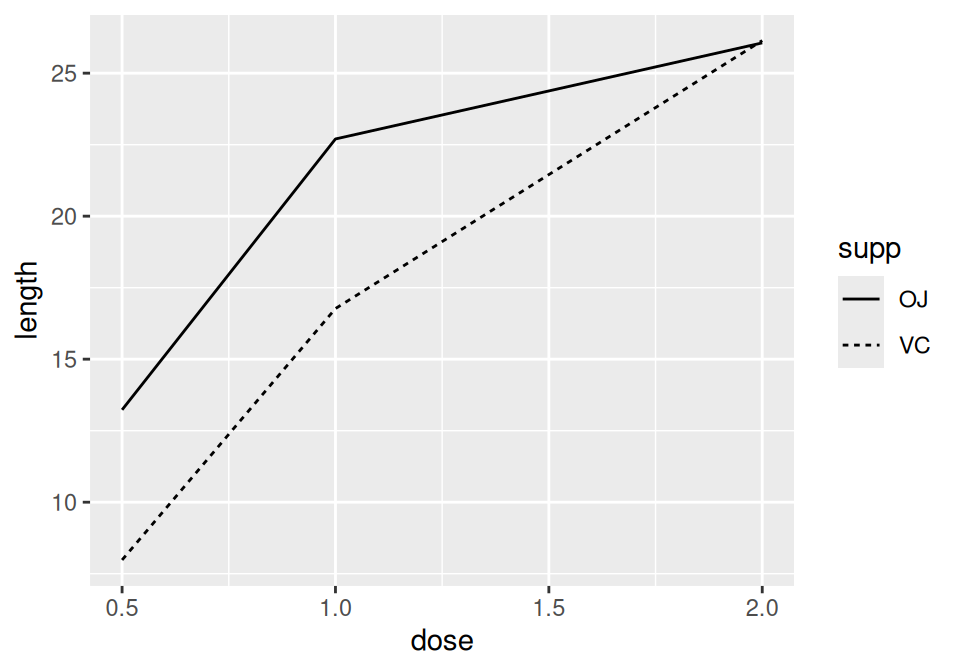Figure 4.6: A variable mapped to colour (left); A variable mapped to linetype (right)

### 4.3.3 Discussion

The `tg` data has three columns, including the factor `supp`, which we mapped to `colour` and `linetype`:

``````tg
#>   supp dose length
#> 1   OJ  0.5  13.23
#> 2   OJ  1.0  22.70
#> 3   OJ  2.0  26.06
#> 4   VC  0.5   7.98
#> 5   VC  1.0  16.77
#> 6   VC  2.0  26.14``````

Note

If the x variable is a factor, you must also tell ggplot to group by that same variable, as described below.

Line graphs can be used with a continuous or categorical variable on the x-axis. Sometimes the variable mapped to the x-axis is conceived of as being categorical, even when it’s stored as a number. In the example here, there are three values of dose: 0.5, 1.0, and 2.0. You may want to treat these as categories rather than values on a continuous scale. To do this, convert `dose` to a factor (Figure 4.7):

``````ggplot(tg, aes(x = factor(dose), y = length, colour = supp, group = supp)) +
geom_line()``````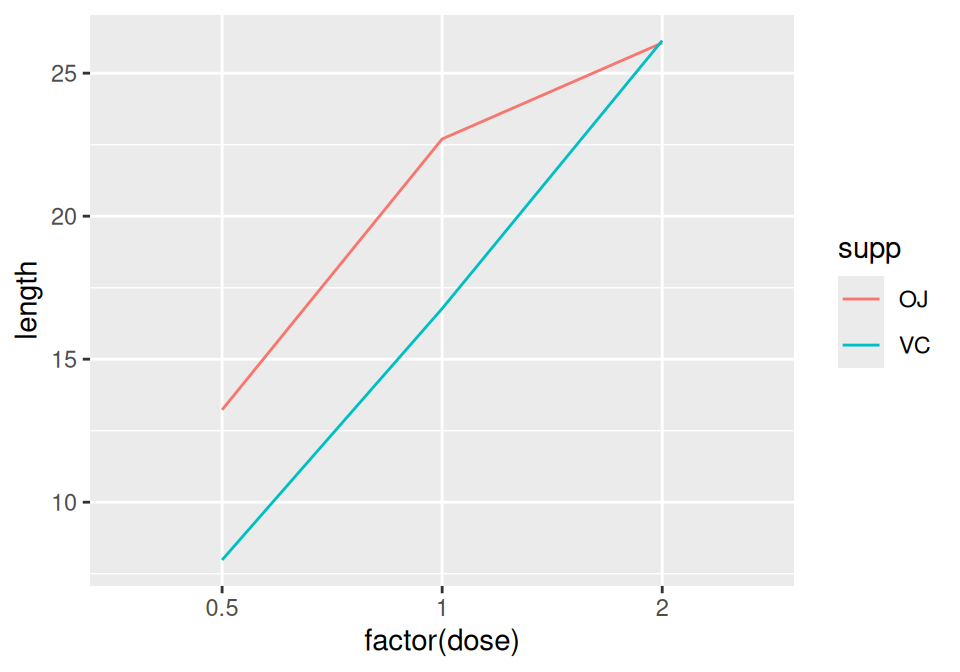Figure 4.7: Line graph with continuous x variable converted to a factor

Notice the use of `group = supp`. Without this statement, ggplot won’t know how to group the data together to draw the lines, and it will give an error:

``````ggplot(tg, aes(x = factor(dose), y = length, colour = supp)) + geom_line()
#> geom_path: Each group consists of only one observation. Do you need to
#> adjust the group aesthetic?``````

Another common problem when the incorrect grouping is used is that you will see a jagged sawtooth pattern, as in Figure 4.8:

``````ggplot(tg, aes(x = dose, y = length)) +
geom_line()``````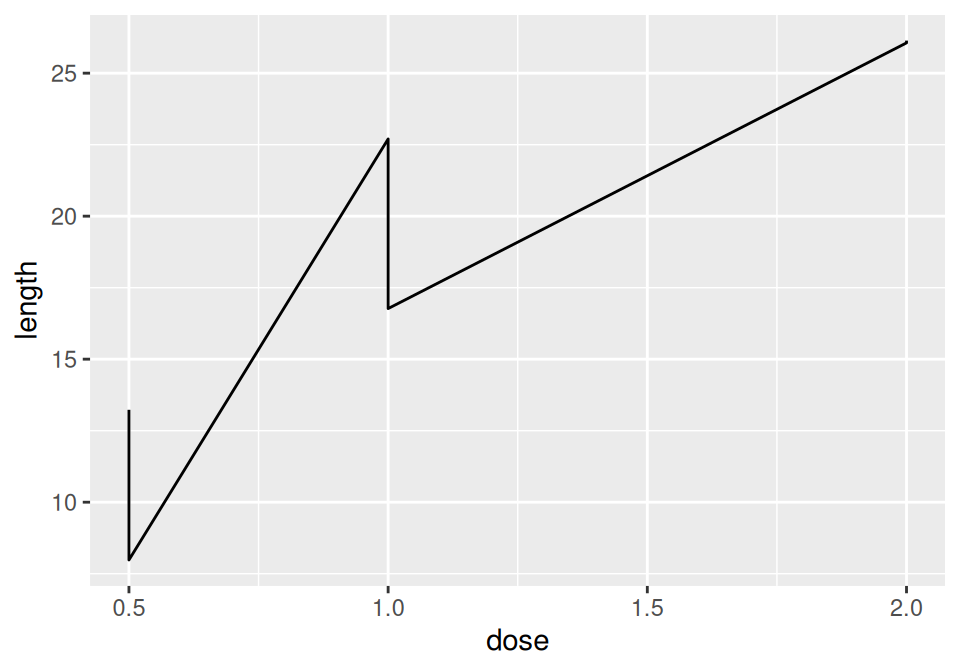Figure 4.8: A sawtooth pattern indicates improper grouping

This happens because there are multiple data points at each y location, and ggplot thinks they’re all in one group. The data points for each group are connected with a single line, leading to the sawtooth pattern. If any discrete variables are mapped to aesthetics like colour or linetype, they are automatically used as grouping variables. But if you want to use other variables for grouping (that aren’t mapped to an aesthetic), they should be used with group.

Note

When in doubt, if your line graph looks wrong, try explicitly specifying the grouping variable with group. It’s common for problems to occur with line graphs because ggplot is unsure of how the variables should be grouped.

If your plot has points along with the lines, you can also map variables to properties of the points, such as shape and fill (Figure 4.9):

``````ggplot(tg, aes(x = dose, y = length, shape = supp)) +
geom_line() +
geom_point(size = 4)  # Make the points a little larger

ggplot(tg, aes(x = dose, y = length, fill = supp)) +
geom_line() +
geom_point(size = 4, shape = 21)  # Also use a point with a color fill``````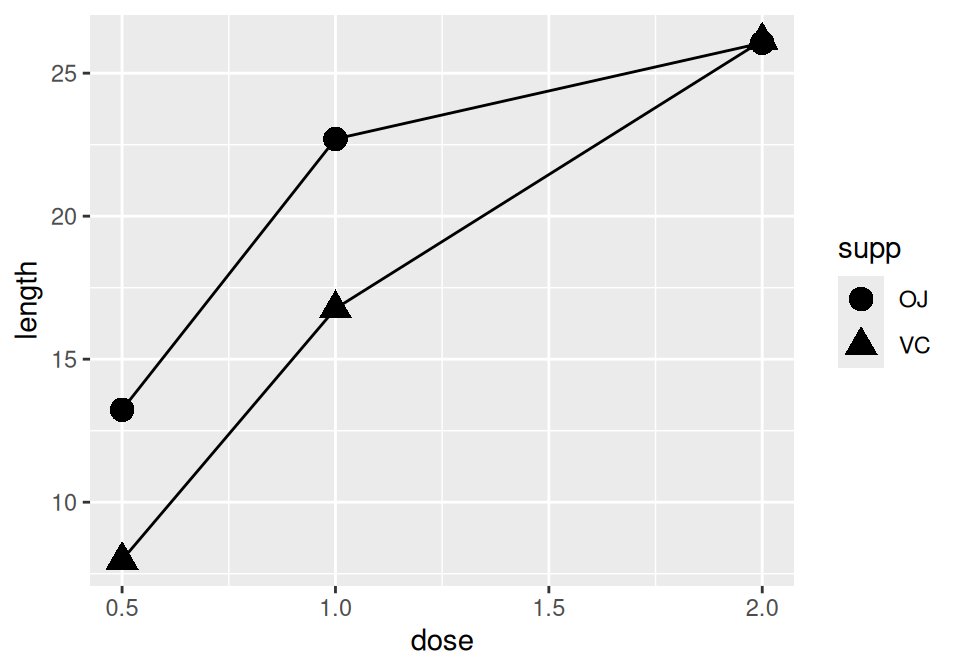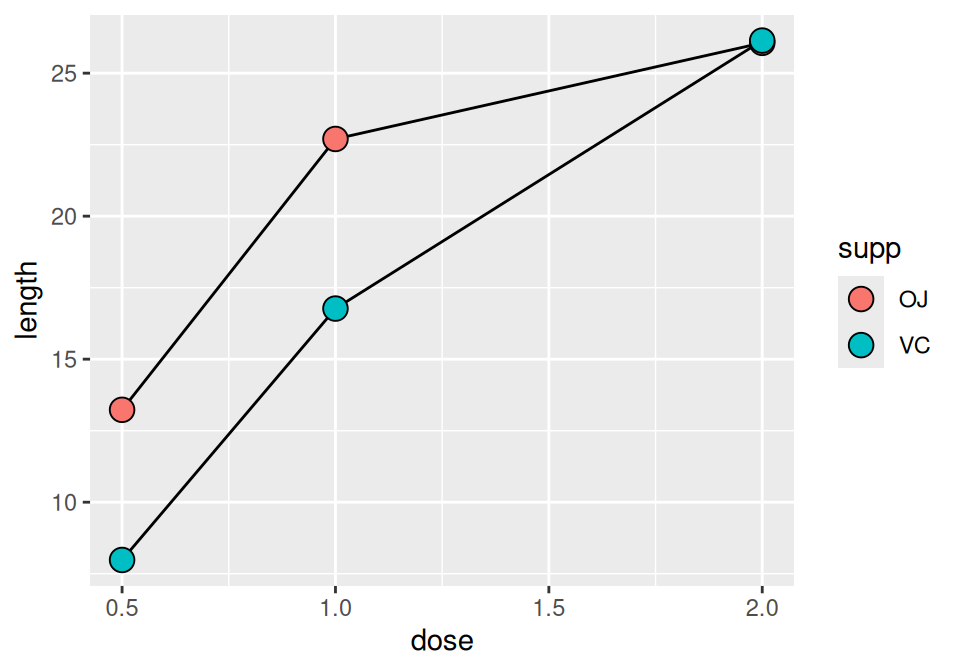Figure 4.9: Line graph with different shapes (left); With different colors (right)

Sometimes points will overlap. In these cases, you may want to dodge them, which means their positions will be adjusted left and right (Figure 4.10). When doing so, you must also dodge the lines, or else only the points will move and they will be misaligned. You must also specify how far they should move when dodged:

``````ggplot(tg, aes(x = dose, y = length, shape = supp)) +
geom_line(position = position_dodge(0.2)) +           # Dodge lines by 0.2
geom_point(position = position_dodge(0.2), size = 4)  # Dodge points by 0.2``````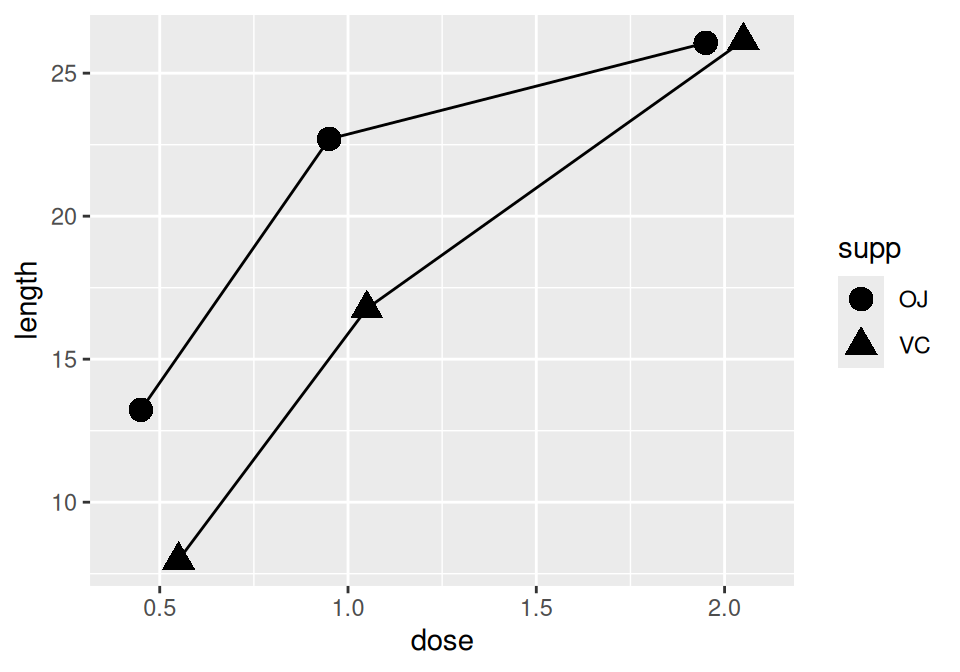Figure 4.10: Dodging to avoid overlapping points

## 4.4 Changing the Appearance of Lines

### 4.4.1 Problem

You want to change the appearance of the lines in a line graph.

### 4.4.2 Solution

The type of line (solid, dashed, dotted, etc.) is set with `linetype`, the thickness (in mm) with `size`, and the color of the line with `colour` (or `color`).

These properties can be set (as shown in Figure 4.11) by passing them values in the call to `geom_line()`:

``````ggplot(BOD, aes(x = Time, y = demand)) +
geom_line(linetype = "dashed", size = 1, colour = "blue")``````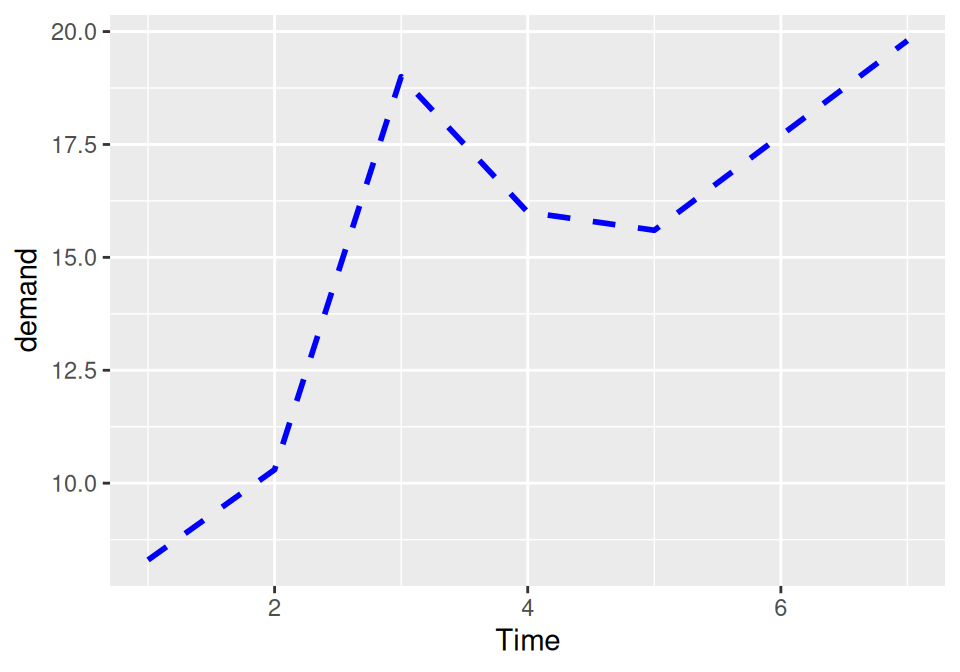Figure 4.11: Line graph with custom linetype, size, and colour

If there is more than one line, setting the aesthetic properties will affect all of the lines. On the other hand, mapping variables to the properties, as we saw in Recipe 4.3, will result in each line looking different. The default colors aren’t the most appealing, so you may want to use a different palette, as shown in Figure 4.12, by using `scale_colour_brewer()` or `scale_colour_manual()`:

``````library(gcookbook)  # Load gcookbook for the tg data set

ggplot(tg, aes(x = dose, y = length, colour = supp)) +
geom_line() +
scale_colour_brewer(palette = "Set1")``````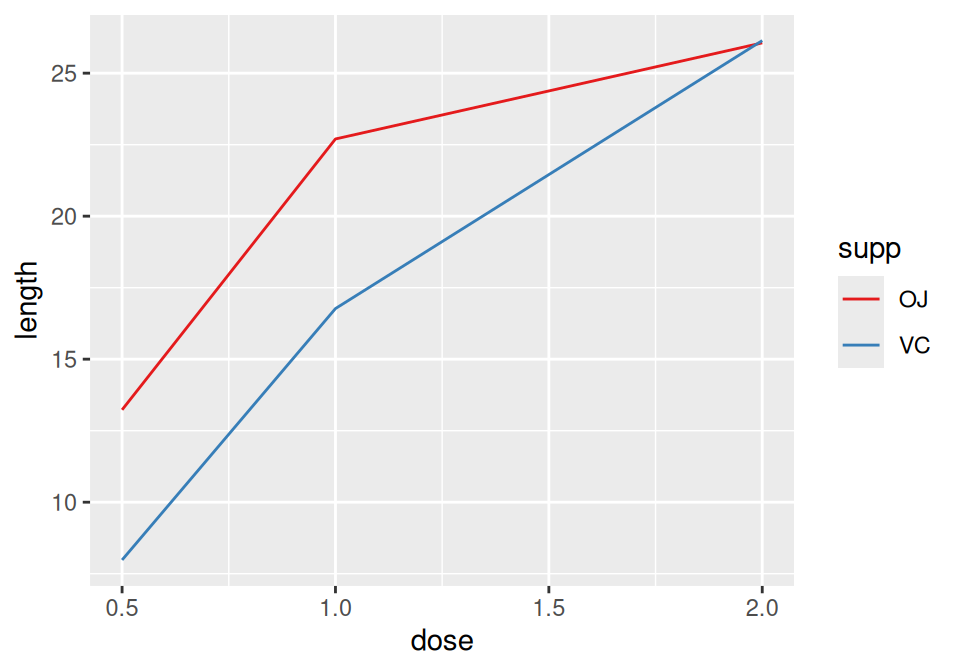Figure 4.12: Using a palette from RColorBrewer

### 4.4.3 Discussion

To set a single constant color for all the lines, specify colour outside of `aes()`. The same works for size, linetype, and point shape (Figure 4.13). You may also have to specify the grouping variable:

``````# If both lines have the same properties, you need to specify a variable to
# use for grouping
ggplot(tg, aes(x = dose, y = length, group = supp)) +
geom_line(colour = "darkgreen", size = 1.5)

# Since supp is mapped to colour, it will automatically be used for grouping
ggplot(tg, aes(x = dose, y = length, colour = supp)) +
geom_line(linetype = "dashed") +
geom_point(shape = 22, size = 3, fill = "white")``````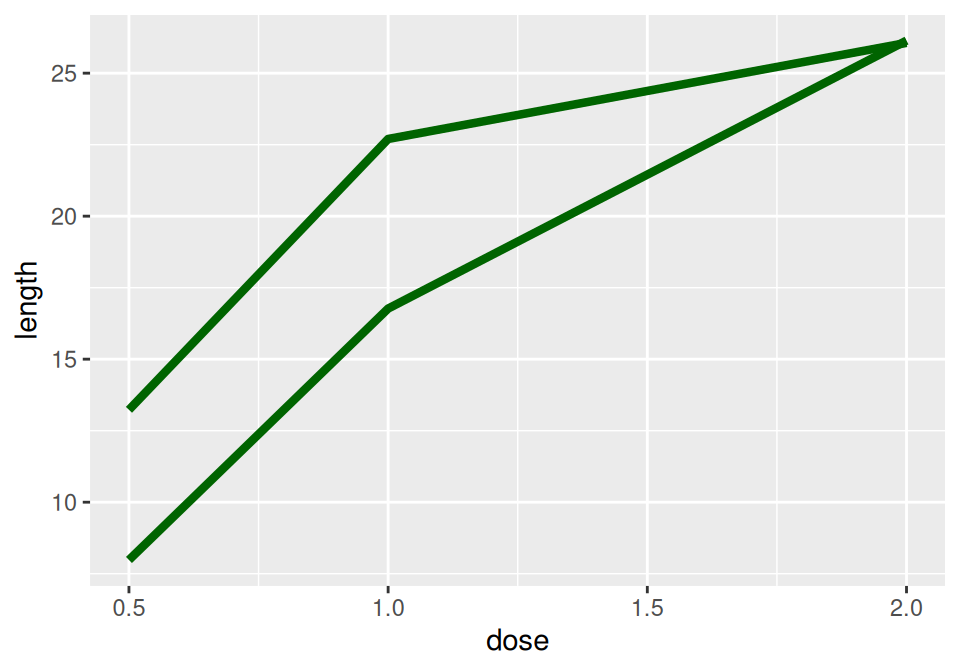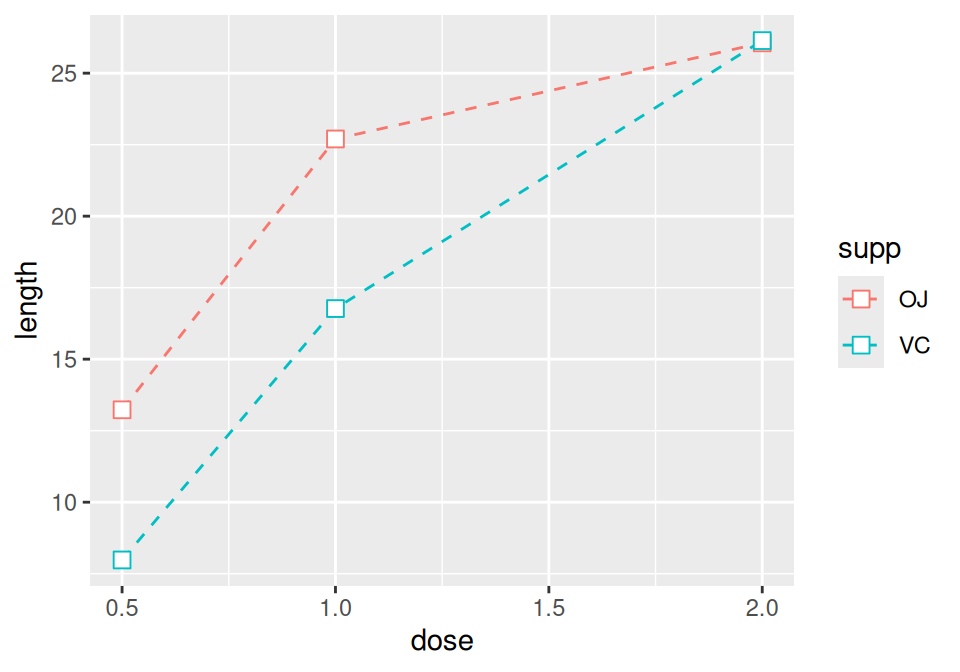Figure 4.13: Line graph with constant size and color (left); With `supp` mapped to `colour`, and with points added (right)

## 4.5 Changing the Appearance of Points

### 4.5.1 Problem

You want to change the appearance of the points in a line graph.

### 4.5.2 Solution

In `geom_point()`, set the `size`, `shape`, `colour`, and/or `fill` outside of `aes()` (the result is shown in Figure 4.14):

``````ggplot(BOD, aes(x = Time, y = demand)) +
geom_line() +
geom_point(size = 4, shape = 22, colour = "darkred", fill = "pink")``````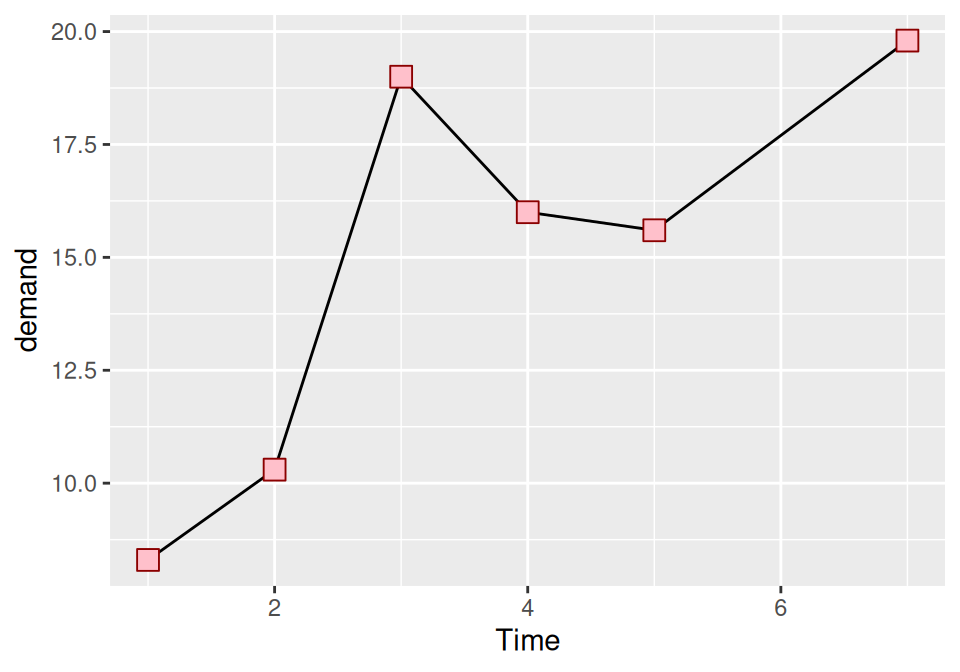Figure 4.14: Points with custom size, shape, color, and fill

### 4.5.3 Discussion

The default shape for points is a solid circle, the default size is 2, and the default colour is black. The fill color is relevant only for some point shapes (numbered 21–25), which have separate outline and fill colors (see Recipe 5.3 for a chart of shapes). The fill color is typically `NA`, or empty; you can fill it with white to get hollow-looking circles, as shown in Figure 4.15:

``````ggplot(BOD, aes(x = Time, y = demand)) +
geom_line() +
geom_point(size = 4, shape = 21, fill = "white")``````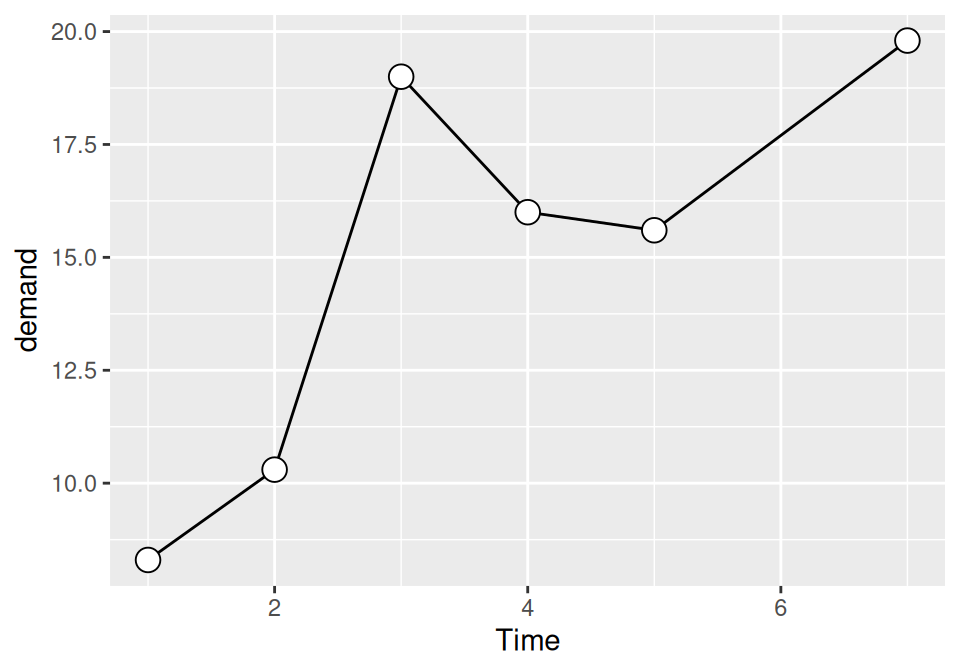Figure 4.15: Points with a white fill

If the points and lines have different colors, you should specify the points after the lines, so that they are drawn on top. Otherwise, the lines will be drawn on top of the points.

For multiple lines, we saw in Recipe 4.3 how to draw differently colored points for each group by mapping variables to aesthetic properties of points, inside of `aes()`. The default colors are not very appealing, so you may want to use a different palette, using `scale_colour_brewer()` or `scale_colour_manual()`. To set a single constant shape or size for all the points, as in Figure 4.16, specify shape or size outside of `aes()`:

``````library(gcookbook)  # Load gcookbook for the tg data set

# Save the position_dodge specification because we'll use it multiple times
pd <- position_dodge(0.2)

ggplot(tg, aes(x = dose, y = length, fill = supp)) +
geom_line(position = pd) +
geom_point(shape = 21, size = 3, position = pd) +
scale_fill_manual(values = c("black","white"))``````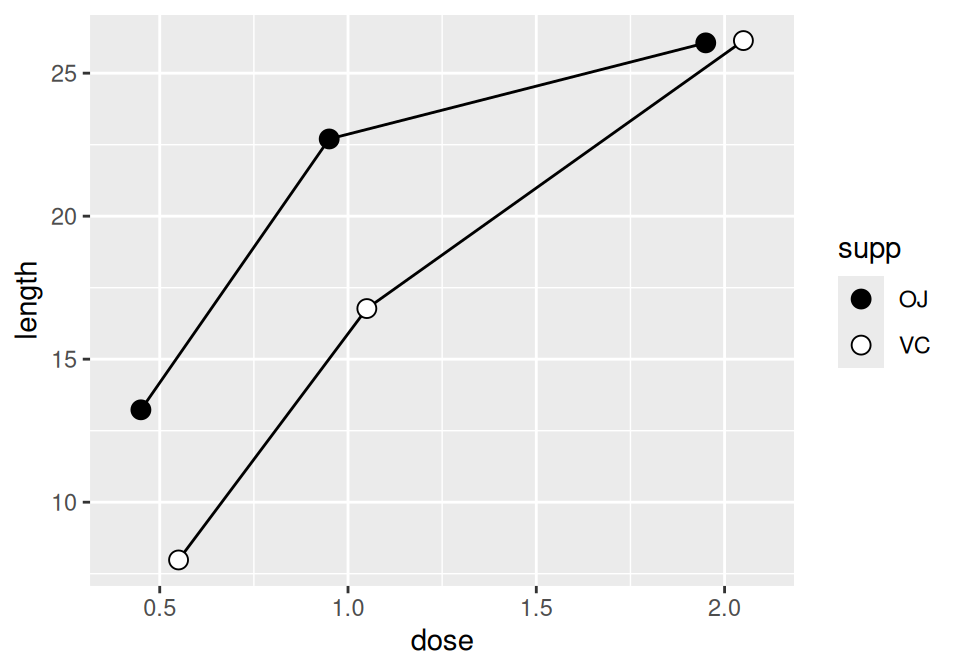Figure 4.16: Line graph with manually specified fills of black and white, and a slight dodge

See Recipe 5.3 for more on using different shapes, and Chapter 12 for more about colors.

## 4.6 Making a Graph with a Shaded Area

### 4.6.1 Problem

You want to make a graph with a shaded area.

### 4.6.2 Solution

Use `geom_area()` to get a shaded area, as in Figure 4.17:

``````# Convert the sunspot.year data set into a data frame for this example
sunspotyear <- data.frame(
Year     = as.numeric(time(sunspot.year)),
Sunspots = as.numeric(sunspot.year)
)

ggplot(sunspotyear, aes(x = Year, y = Sunspots)) +
geom_area()``````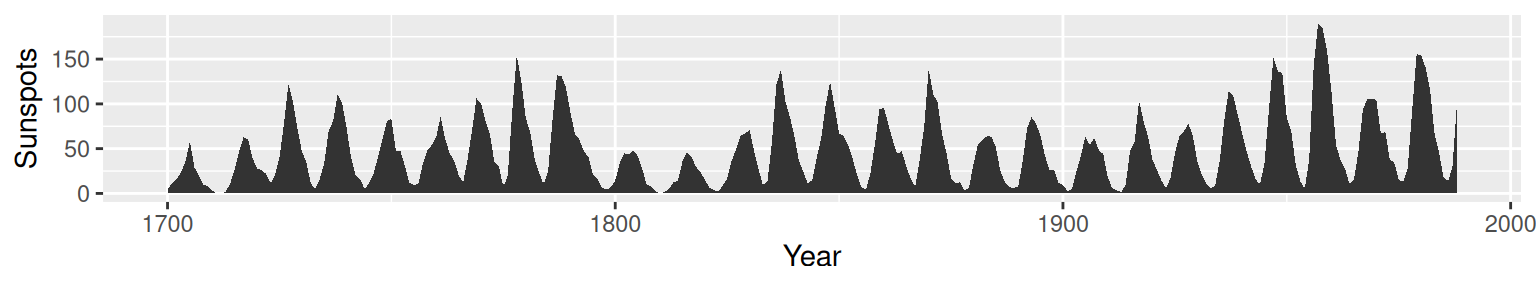Figure 4.17: Graph with a shaded area

### 4.6.3 Discussion

By default, the area will be filled with a very dark grey and will have no outline. The color can be changed by setting `fill`. In the following example, we’ll set it to `"blue"`, and we’ll also make it 80% transparent by setting `alpha` to 0.2. This makes it possible to see the grid lines through the area, as shown in Figure 4.18. We’ll also add an outline, by setting colour:

``````ggplot(sunspotyear, aes(x = Year, y = Sunspots)) +
geom_area(colour = "black", fill = "blue", alpha = .2)``````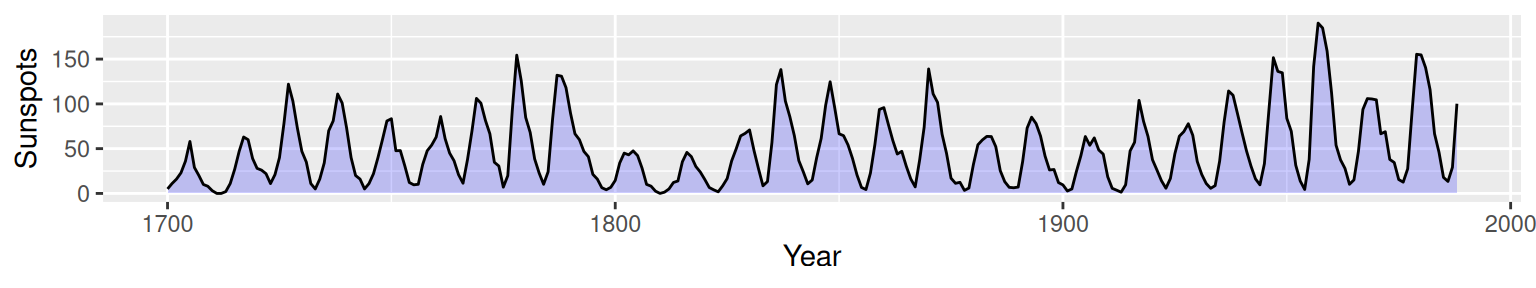Figure 4.18: Graph with a semitransparent shaded area and an outline

Having an outline around the entire area might not be desirable, because it puts a vertical line at the beginning and end of the shaded area, as well as one along the bottom. To avoid this issue, we can draw the area without an outline (by not specifying colour), and then layer a `geom_line()` on top, as shown in Figure 4.19:

``````ggplot(sunspotyear, aes(x = Year, y = Sunspots)) +
geom_area(fill = "blue", alpha = .2) +
geom_line()``````Figure 4.19: Line graph with a line just on top, using `geom_line()`

See Chapter 12 for more on choosing colors.

## 4.7 Making a Stacked Area Graph

### 4.7.1 Problem

You want to make a stacked area graph.

### 4.7.2 Solution

Use `geom_area()` andmap a factor to fill (Figure 4.20):

``````library(gcookbook) # Load gcookbook for the uspopage data set

ggplot(uspopage, aes(x = Year, y = Thousands, fill = AgeGroup)) +
geom_area()``````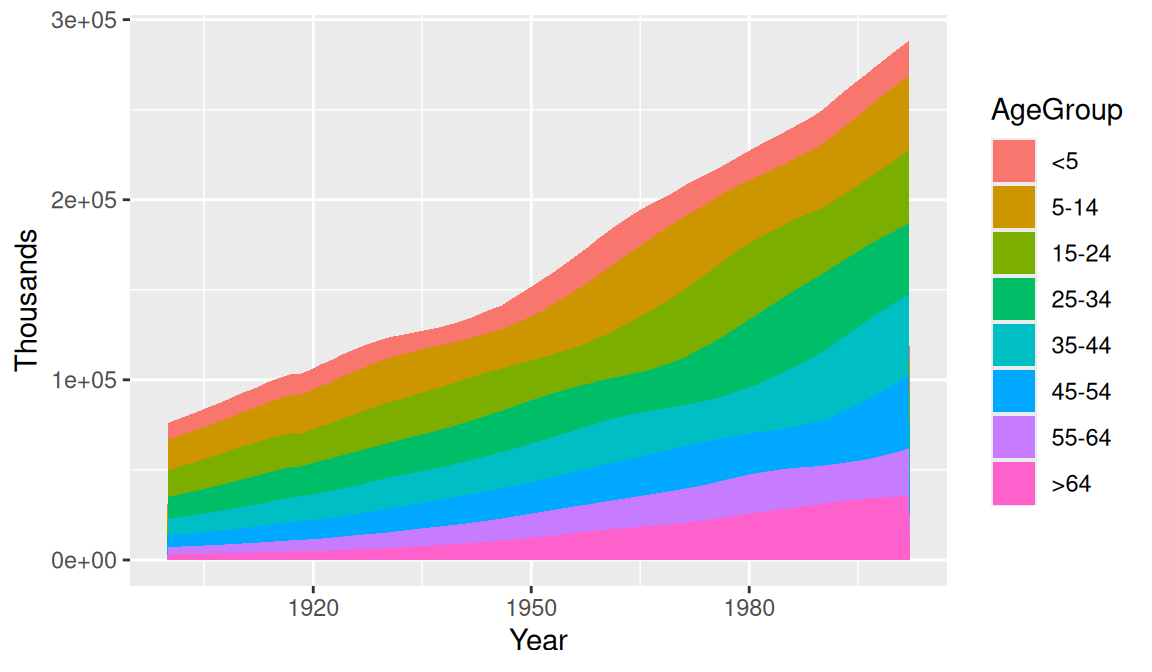Figure 4.20: Stacked area graph

### 4.7.3 Discussion

The sort of data that is plotted with a stacked area chart is often provided in a wide format, but ggplot requires data to be in long format. To convert it, see Recipe 15.19.

In the example here, we used the `uspopage` data set:

``````uspopage
#>     Year AgeGroup Thousands
#> 1   1900       <5      9181
#> 2   1900     5-14     16966
#> 3   1900    15-24     14951
#>  ...<818 more rows>...
#> 822 2002    45-54     40084
#> 823 2002    55-64     26602
#> 824 2002      >64     35602``````

This version of the chart (Figure 4.21) changes the palette to a range of blues and adds thin (`size = .2`) lines between each area. It also makes the filled areas semitransparent (`alpha = .4`), so that it is possible to see the grid lines through them:

``````ggplot(uspopage, aes(x = Year, y = Thousands, fill = AgeGroup)) +
geom_area(colour = "black", size = .2, alpha = .4) +
scale_fill_brewer(palette = "Blues")``````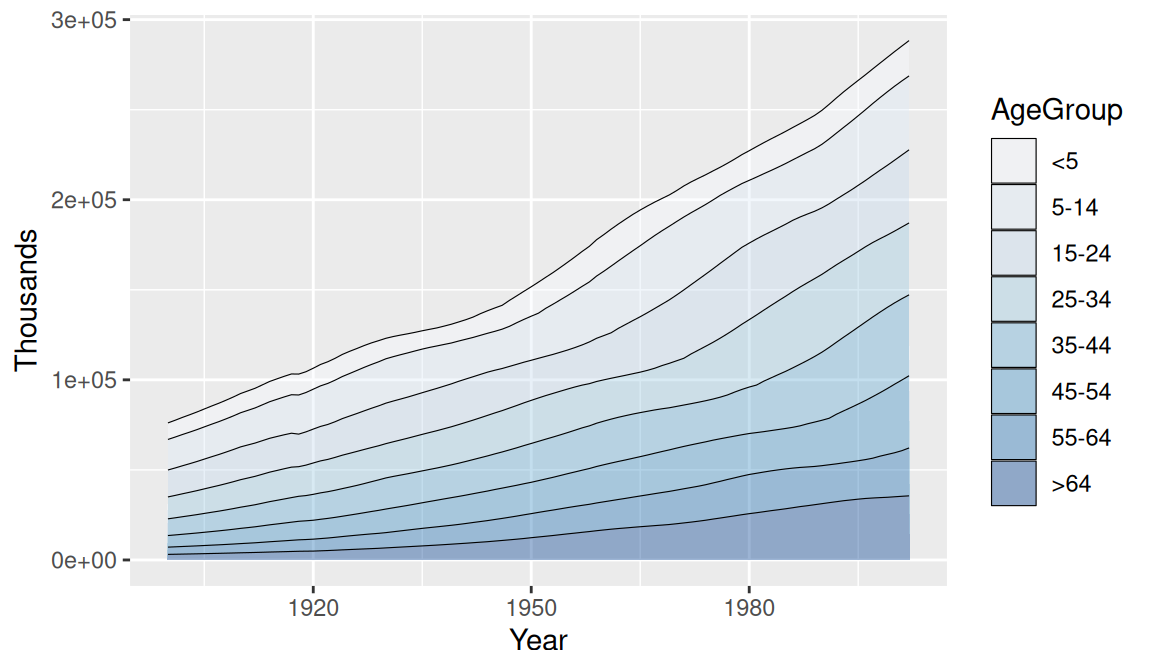Figure 4.21: Reversed legend order, lines, and a different palette

Since each filled area is drawn with a polygon, the outline includes the left and right sides. This might be distracting or misleading. To get rid of it (Figure 4.22), first draw the stacked areas without an outline (by leaving `colour` as the default `NA` value), and then add a `geom_line()` on top:

``````ggplot(uspopage, aes(x = Year, y = Thousands, fill = AgeGroup, order = dplyr::desc(AgeGroup))) +
geom_area(colour = NA, alpha = .4) +
scale_fill_brewer(palette = "Blues") +
geom_line(position = "stack", size = .2)``````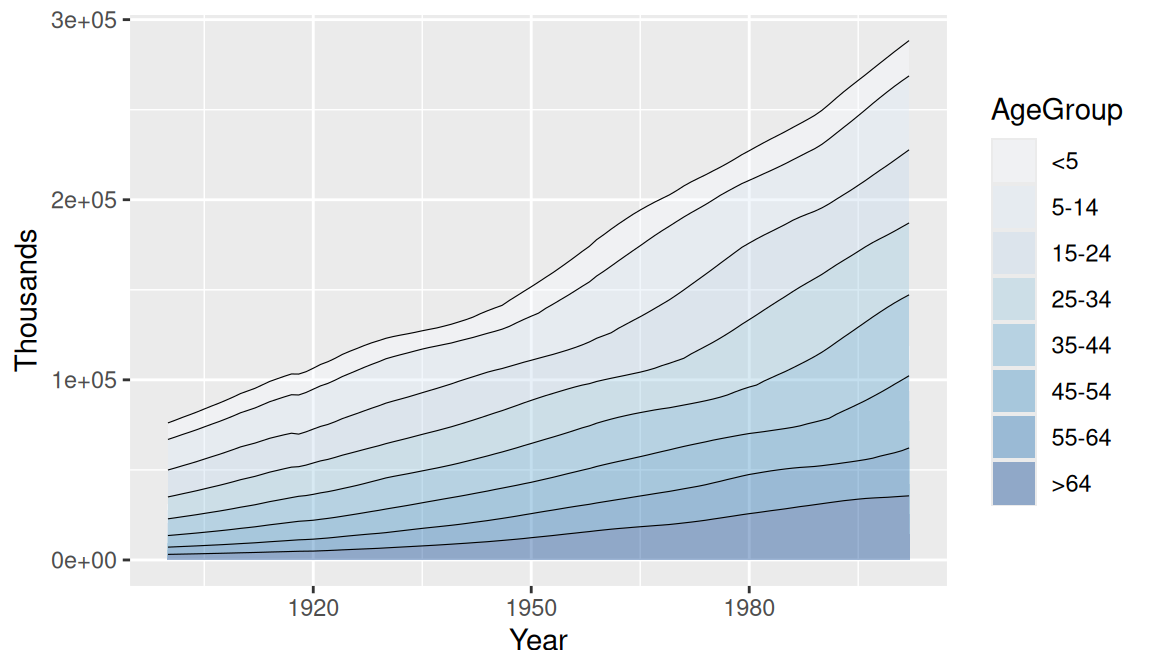Figure 4.22: No lines on the left and right of the graph

See Recipe 15.19 for more on converting data from wide to long format.

See Chapter 12 for more on choosing colors.

## 4.8 Making a Proportional Stacked Area Graph

### 4.8.1 Problem

You want to make a stacked area graph with the overall height scaled to a constant value.

### 4.8.2 Solution

Use `geom_area(position = "fill")`, as in Figure 4.23, left:

``````ggplot(uspopage, aes(x = Year, y = Thousands, fill = AgeGroup)) +
geom_area(position = "fill", colour = "black", size = .2, alpha = .4) +
scale_fill_brewer(palette = "Blues")``````

### 4.8.3 Discussion

With `position="fill"`, the y values will be scaled to go from 0 to 1. To print the labels as percentages, use `scale_y_continuous(labels = scales::percent)`, as in Figure 4.23, right:

``````ggplot(uspopage, aes(x = Year, y = Thousands, fill = AgeGroup)) +
geom_area(position = "fill", colour = "black", size = .2, alpha = .4) +
scale_fill_brewer(palette = "Blues") +
scale_y_continuous(labels = scales::percent)``````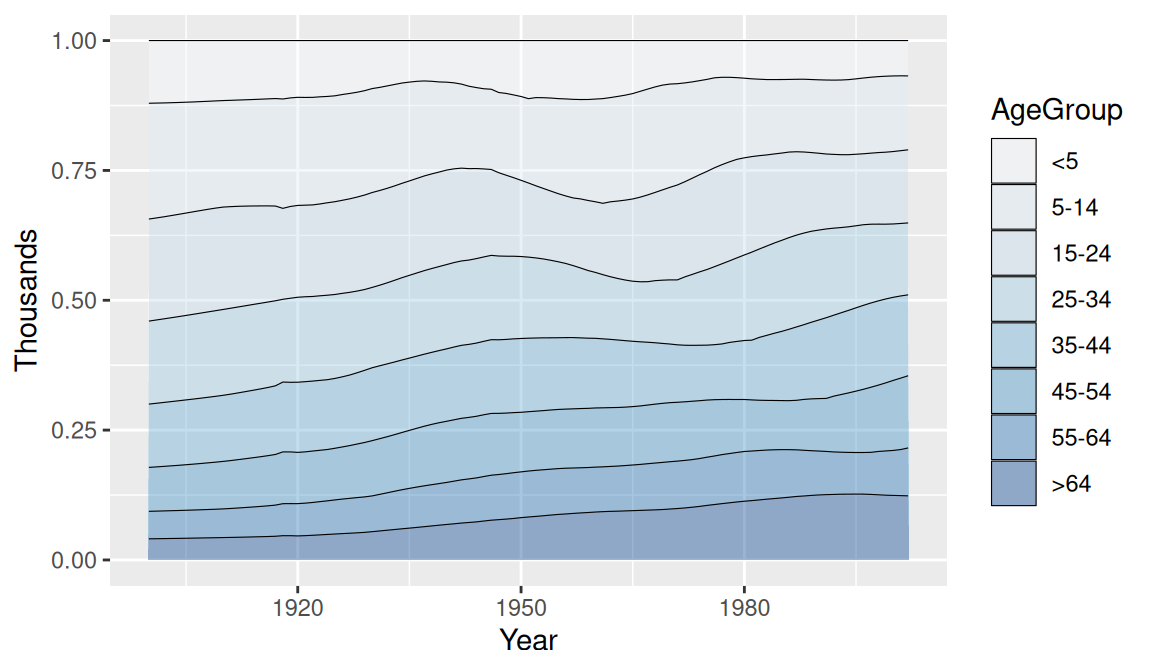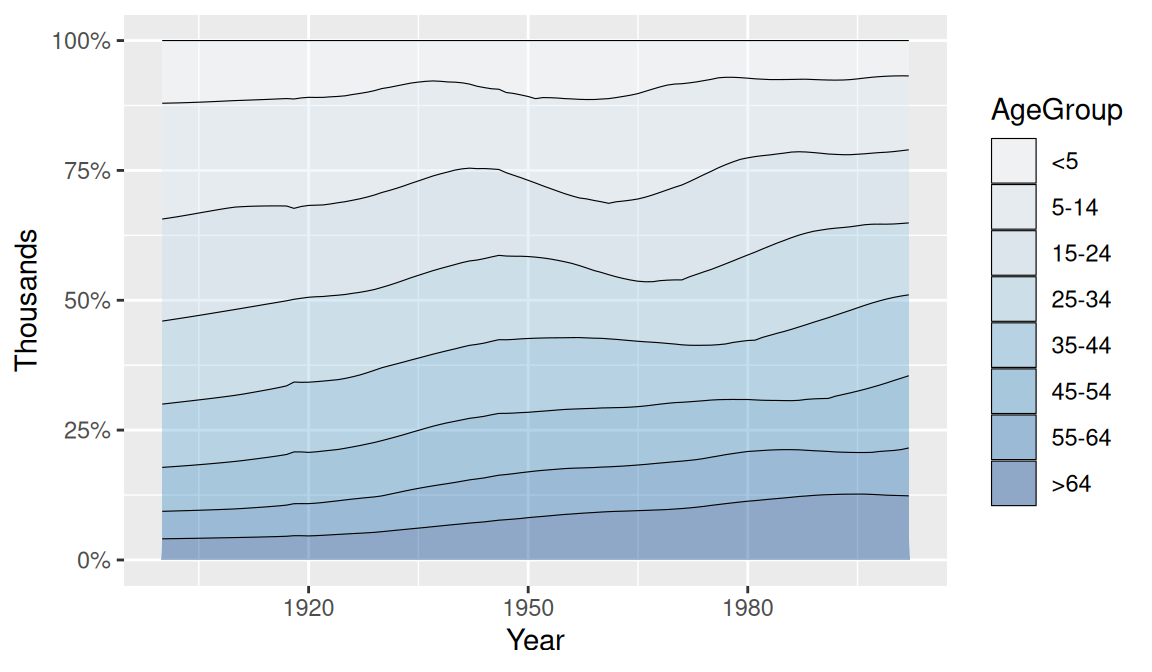Figure 4.23: A proportional stacked area graph

Creating stacked bar graph is done in a similar way. See Recipe 3.8 for information about computing the percentages separately.

For more on summarizing data by groups, see Recipe 15.17.

## 4.9 Adding a Confidence Region

### 4.9.1 Problem

You want to add a confidence region to a graph.

### 4.9.2 Solution

Use `geom_ribbon()` and map values to `ymin` and `ymax`.

In the `climate` data set, `Anomaly10y` is a 10-year running average of the deviation (in Celsius) from the average 1950–1980 temperature, and `Unc10y` is the 95% confidence interval. We’ll set `ymax` and `ymin` to `Anomaly10y` plus or minus `Unc10y` (Figure 4.24):

``````library(gcookbook) # Load gcookbook for the climate data set
library(dplyr)

# Grab a subset of the climate data
climate_mod <- climate %>%
filter(Source == "Berkeley") %>%
select(Year, Anomaly10y, Unc10y)

climate_mod
#>     Year Anomaly10y Unc10y
#> 1   1800     -0.435  0.505
#> 2   1801     -0.453  0.493
#> 3   1802     -0.460  0.486
#>  ...<199 more rows>...
#> 203 2002      0.856  0.028
#> 204 2003      0.869  0.028
#> 205 2004      0.884  0.029

ggplot(climate_mod, aes(x = Year, y = Anomaly10y)) +
geom_ribbon(aes(ymin = Anomaly10y - Unc10y, ymax = Anomaly10y + Unc10y), alpha = 0.2) +
geom_line()``````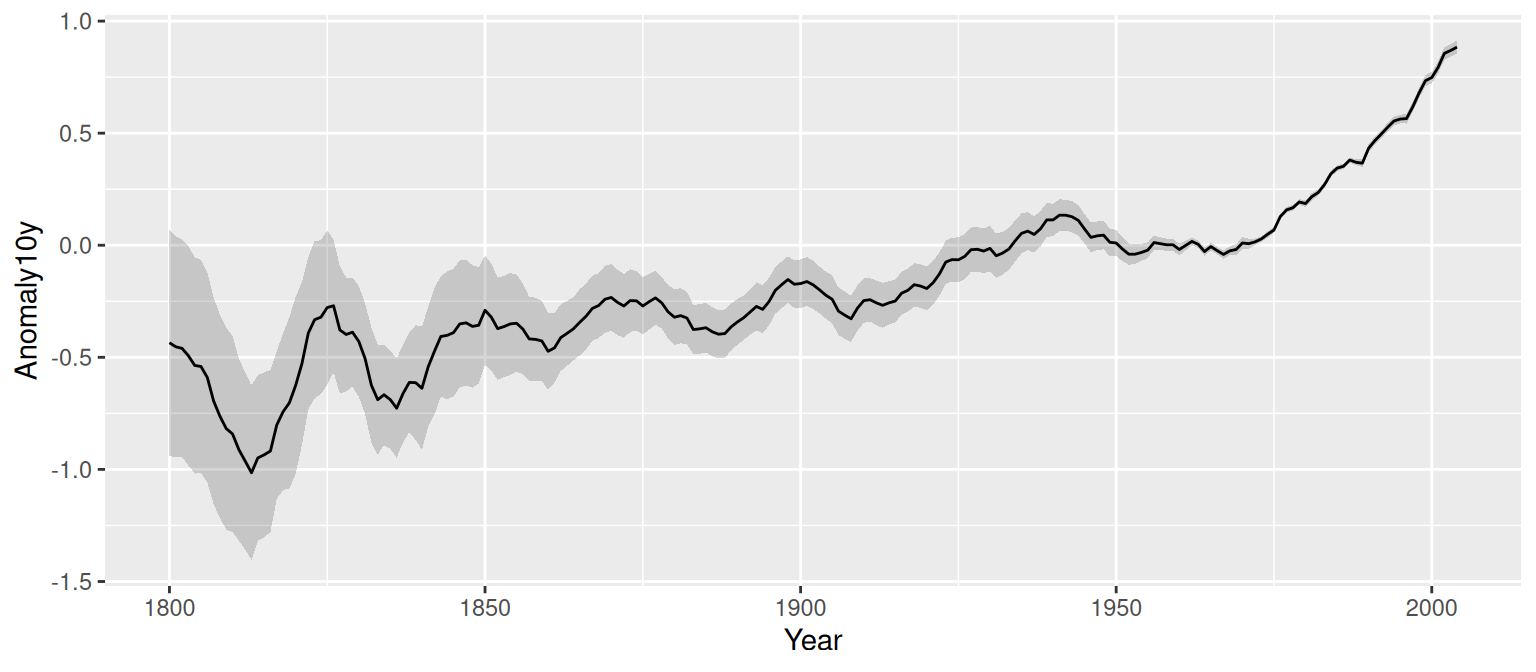Figure 4.24: A line graph with a shaded confidence region

The shaded region is actually a very dark grey, but it is mostly transparent. The transparency is set with `alpha = 0.2`, which makes it 80% transparent.

### 4.9.3 Discussion

Notice that the `geom_ribbon()` comes before `geom_line()`, so that the line is drawn on top of the shaded region. If the reverse order were used, the shaded region could obscure the line. In this particular case that wouldn’t be a problem since the shaded region is mostly transparent, but it would be a problem if the shaded region were opaque.

Instead of a shaded region, you can also use dotted lines to represent the upper and lower bounds (Figure 4.25):

``````# With a dotted line for upper and lower bounds
ggplot(climate_mod, aes(x = Year, y = Anomaly10y)) +
geom_line(aes(y = Anomaly10y - Unc10y), colour = "grey50", linetype = "dotted") +
geom_line(aes(y = Anomaly10y + Unc10y), colour = "grey50", linetype = "dotted") +
geom_line()``````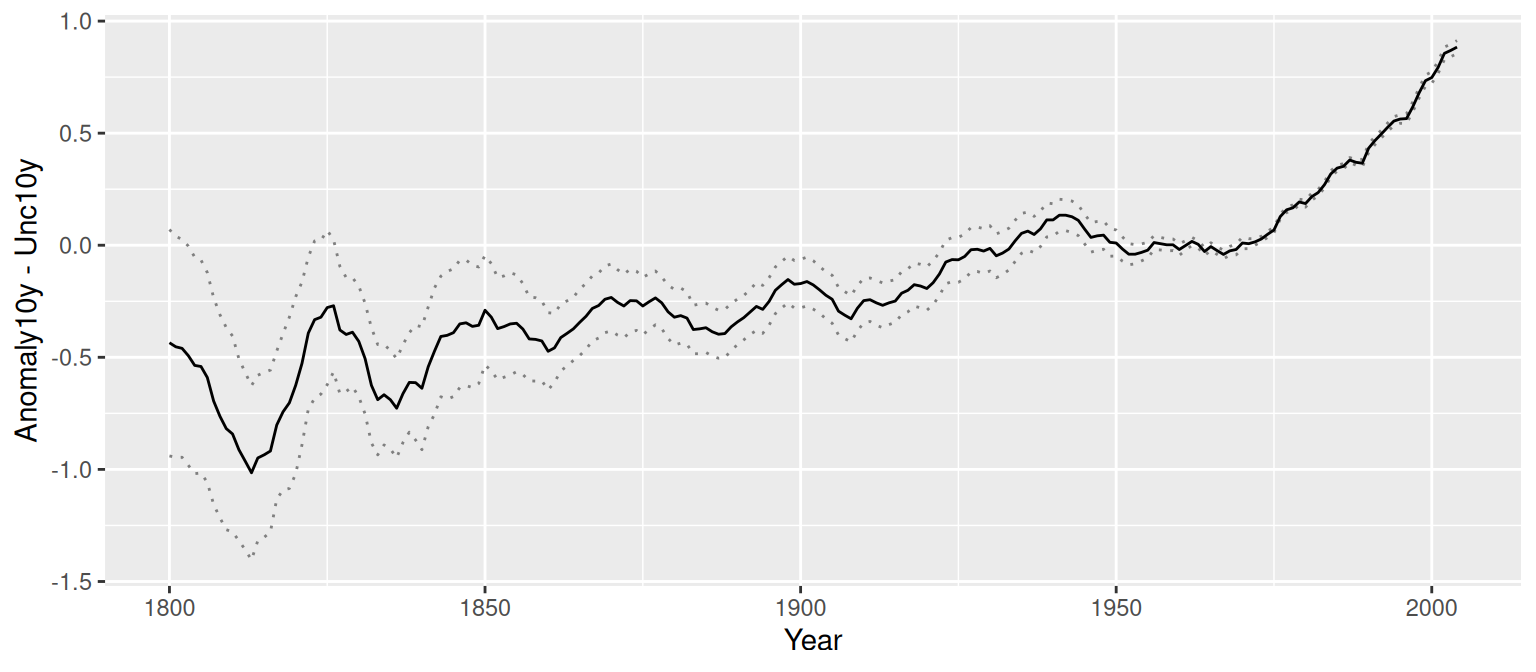Figure 4.25: A line graph with dotted lines representing a confidence region

Shaded regions can represent things other than confidence regions, such as the difference between two values, for example.

In the area graphs in Recipe 4.7, the y range of the shaded area goes from 0 to y. Here, it goes from `ymin` to `ymax`.